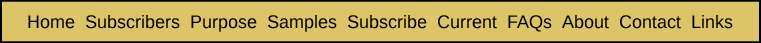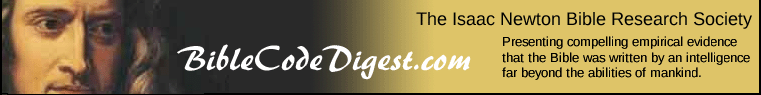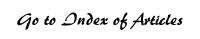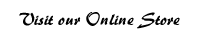The Probability of a Given ELS, Which Only Occurs Once, of Crossing a Specific Section of Text Appendix Three from Breakthrough: Encountering the Reality of the Bible Codes We will now look at how to calculate the probability that a given ELS will intersect a specific section of text within a larger body of text. For simplicity's sake, we will assume that this ELS only occurs once within the larger text. Once we calculate this probability, we can determine the likelihood of a crossing given any whole number of occurrences of the ELS. This will be covered in Appendix Five. In the previous appendices, we have covered some of the key pieces of information we will use in solving this problem. The most important is a precise determination of the total number of possible ELSs of a given interval i that may be formed within a text of length T. This is given by Formula 1B, which is repeated below for ease of reference.It should be remembered that this only gives the number of possible forward ELSs or the number of possible backward ELSs. The probability we are seeking to determine will be the total number of ELSs that cross the given section of text (of width W) divided by the total number of all possible ELSs which can occur within the larger text. We will first make this derivation using a very specific example, and will then generalize it with algebraic formulae and notation. First, let us go back to our earlier example of the first 50 letters of text in Lincoln's Gettysburg Address. We show this section of text below, with the numerical position of every fifth letter noted underneath. Every fifth letter is also shown in bold type.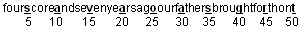We will focus on the small section of text which consists of the 30th through the 32nd letters of the above larger text. In the above text, these positions are occupied by the letters a, t and h. To emphasize this, we repeat the above text with these letters in caps and a larger font: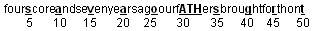We will first count the number of forward ELSs of length (L) of three skips and interval (i) that intersect with this small section of text, which we will denote by the numbers of their positions relative to the first letter of the whole text (30,31,32). Suppose we start with the case of ELSs with intervals of 1. The ELSs with 4 letters (3 skips) that can overlap the above section of text are: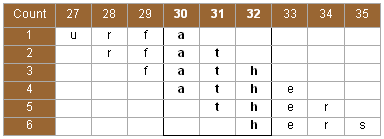As shown above, the six possible ELSs are: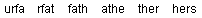They could also be denoted as: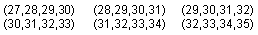What impact do the width (W) of the section of text and the length (L) of the ELS have on the number of ELSs of interval 1 that overlap the given section? Let's look at what happens when we increase L by 2.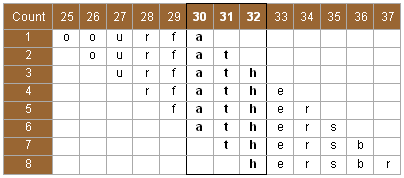The number of possible ELS's increases by two to eight. Now the first three ELSs are similar in both cases because they each end within the (30,31,32) section of the text. What is different is the number of ELSs that end in spaces beyond the end of the (30,31,32) section. When L = 3, there are three ELSs that end beyond the given section, and when L = 5, there are five such ELSs. The number ending within the section itself is simply the width of the section (W), because each ending letter coincides with one letter in the section. The number of ELSs ending beyond the given section is L, since the last eligible ELS must still have one letter in the given section. That limits the number of eligible ELSs to one less than the given number of letters in the ELS itself, which is the same as the number of skips (L). Combining the formulas for each type of ELS (i.e., those whose ending letter falls within the section and those whose ending letter falls beyond the given section) gives us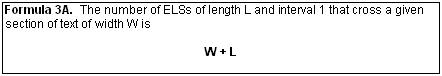Next, let us look at all of the ELSs with three skips and with an interval of 2 that overlap with the (30,31,32) section of text.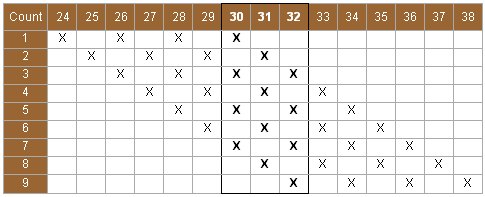Notice that there are nine possible ELSs of interval 2, which is three more than the six possible ELSs of interval 1. It may be observed that there is no difference in the number of ELSs ending within the section of text of width W = 3. It is still three, even though the interval has increased from 1 to 2, because there is only one ELS with an ending letter that is one of the letters of the given section of text. What is different is that the number of ELSs ending beyond the given section has increased from three to six, or from L to 2*L. Similarly, the following table lays out all of the ELSs with three skips that have an interval of 3 and that overlap (30,31,32).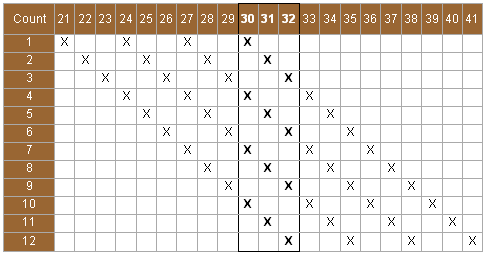Notice that there are 12 possible ELSs of interval 3, which is again three more than the nine ELSs possible with an interval of 2. So, the number of ELSs which end beyond the given section of text has increased from 2L to 3L. The effect of increasing the interval is that it stretches out the degree to which ELSs can extend out beyond the given section of text. The amount by which the ELSs extending beyond the given section of text are stretched is equal to the size of the interval times the number of skips between the ELS letters. Let's consider some patterns that have emerged as we have looked at the three examples above where L was 3 and the intervals were 1, 2 and 3. Suppose we record in a table the numbers of the beginning and ending letters of the first ELS that crosses the given section of text and the numbers of the beginning and ending letters of the last ELS that crosses the section. This gives us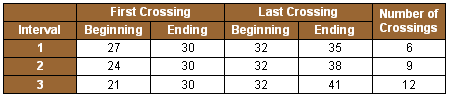Notice that the ending letter of every first crossing is 30. This is by definition, because we are ordering the ELSs starting with those with lower numbered positions. Also, the beginning number of the last ELS to crossing is always 32, again by definition. Next it may be noted that the ending number of the last crossing ELS increases by three as the interval is increased by 1. As we saw above, the increment of 3 is related to the number of skips between the letters of the ELS (L), rather than to the width W of the section of text. We also saw that the number of crossings also increases by three with each increase of one in the interval because L = 3. In each case, we have determined the number of crossing ELSs by counting from the ending letter of the first ELS to the ending letter of the last ELS. In each case the number of crossings is determined by starting with the number position of the ending letter of the last crossing ELS, subtracting the number position of the ending letter of the first crossing ELS, and adding one. In the case of the interval of 1, this gives us 35 – 30 + 1 = 6. Why do we add 1? Suppose we want to know how many numbers there are between, and including, 30 and 35. If we actually write them out and count them we get an answer of 6.If we merely take the difference between 35 and 30 (5), we fall short by 1. If we only take the difference of the two numbers we in effect exclude counting the first number. To do what we are trying to do by subtracting the number position of the last letter from the number position of the first letter, we really should be including 0 in our counting. In the above example, then, we would get the right answer if we subtracted 29 from 35 rather than 30.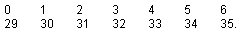Getting the total number of numbers between 30 and 35, including both of these numbers, is really the same as determining the number of numbers between 1 and 6, including both of these. Obviously, if we merely subtract 1 from 6, and get an answer of 5, we are off by one. What we really needed to do was to subtract 0 from 6. A curious thing happens when the interval is increased from 3 to 4. The following table shows the various ELSs of interval 4 that cross the selected section of text. When we come to the fourth ELS, it consists of letters 21, 25, 29 and 33. None of these actually cross the section of text consisting of letters 30, 31 and 32. Instead this ELS "surrounds" the section of text without actually intersecting, or crossing, it. This happens with every fourth ELS. This phenomenon is possible because the interval (4) is greater than the width (3) of the section of text. As a result, the total number of ELSs that cross the given section of text remains at 12 as the interval increases from 3 to 4.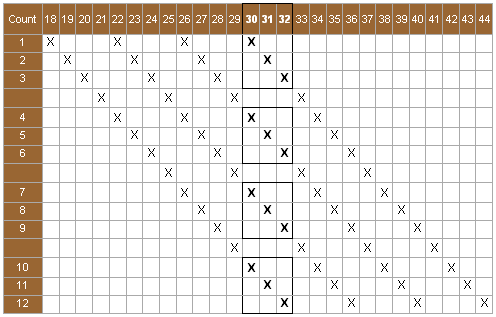When the interval increases from 4 to 5, we again find that the total number of ELSs that cross still remains at 12. This also happens when the interval increases from 5 to 6. However, when the interval increases from 6 to 7, the total number of crossing ELSs drops to nine because some possible ELSs are excluded since they extend beyond the end of the total text (the first 50 letters). All this can be seen in the layouts below. A box indicates a circumstance where the ELS crosses the (30,31,32) section of text.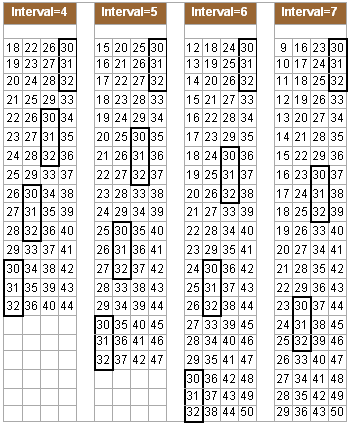Note that three ELSs [(30,37,44,51), (31,38,45,52) and (32,39,46,53)] were excluded because they extend beyond the end of the text. What happens for the next group of possible ELSs? For the ELSs with intervals 8 and 9, the last three possible crossing ELSs are also excluded because they again extend beyond the 50th letter. When we look at the ELSs with an interval of 10, we see that the first crossing ELS was excluded because it would have to extend back before the beginning of the text [(0,10,20,30)]. Furthermore, for the ELSs with an interval of 11, all of the first group of possible crossing ELSs are excluded for the same reason.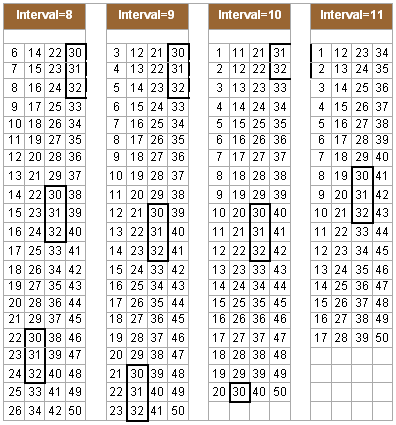It is clear that for ELSs with intervals of 10 or more, some of the crossing ELSs are excluded because they either extend back before the first letter or beyond the 50th letter. Possible ELSs for intervals of 12 through 15 are shown below.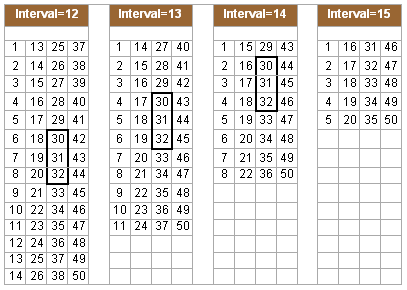Finally, for interval 16, there are only two possible ELSs [(1,17,33,49) and (2,18,34,50)] and neither of these cross the section of text. The following table summarizes what we have determined over the last few pages in counting the number of ELSs that cross the section of text covering the 30th through the 32nd letters. Table 3AThere are several interesting observations that may be made about the above table. We have shown in bold type the number of the letter that is defined by some condition of the specific example we have at hand. For intervals 1 through 6, 30 and 32 are shown in bold because either the first or last ELS is defined as either the first or last number of the specific section of text in the example (i.e., 30 or 32). For intervals 7 through 16, the number 50 is shown in bold because the last ELS possible is defined as the right boundary of the full text of 50 letters. For intervals 10 through 16, the number 1 is shown in bold because the first ELS possible is defined as the left boundary of the full text of 50 letters. The above example shows that there are three regions to consider in terms of calculating the probability of overlap of a given ELS with a specific section of text. Each of these regions will be defined in terms of a range of interval (i) values. We will call the first region R0 because there are no ELSs for intervals in this region which can possibly reach beyond either of the boundaries of the complete text. In other words, no ELS can reach all the way from the given section of text to a letter position beyond either the beginning or the ending of the complete text. In our example, if the interval is any value between 1 and 6, then no ELS will be excluded from our collection because one of its letters might fall beyond either the beginning (position 1) or ending (position 50) of our full text, that is the first 50 letters of the Gettysburg Address. When the interval is 6, however, one of the ELSs begins at position 32 and ends exactly at position 50. This rightmost possible ELS does not extend beyond the ending of our text, however. Similarly, we will call the second region R1 to designate that for interval (i) values in this region, ELSs cannot extend beyond more than one boundary of the complete text. Finally, we will call the third region R2 to reflect the fact that for interval (i) values in this region, one or more ELSs can reach beyond either boundary of the entire text. Let's look at the first interval value of Region R1 where i = 7. According to the table, the last possible overlapping ELS begins at 29 and ends at 50. This ELS is (29,36,43,50). There are, however, three additional ELSs with interval 7 that extend beyond the end of our text of 50 letters. They are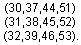These three possible ELSs have been excluded because any letter position above 50 is not possible. What is evident from the above example is that for any given interval (i) the number of crossing ELSs cannot be greater than 12. This maximum is defined by W + (W * L). This is true because the first W crossing ELSs are defined by a letter of the section of text. The remaining crossing ELSs are defined by an ending letter that extends out to the right beyond the section of text, and the number of spaces that such crossing ELSs can extend out beyond the section of text is W * L. However, it is possible for some intervals that some of the ELSs will extend beyond the end of the entire text and so be disqualified — as will those that would stretch out prior to the beginning of the entire text. When we cross over from Region R1 to R2, possible ELSs are disqualified at both ends of the text. As the table shows for an interval of 10, the first allowable ELS begins at 1 and ends at 31. It is (1,11,21,31). We excluded (0,10,20,30) because 0 is outside the range of our entire text. At the other end, we excluded twelve ELSs: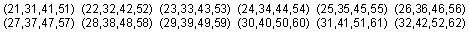This is three more than the nine ELSs we excluded for interval 9 because they extended beyond the end of our text: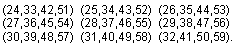Each time we move from one interval to the next one within region R1, we end up excluding three more ELSs than we did for the previous given interval. The fact that we are excluding three more is based on the length of the ELS (three skips). Correspondingly, once we are in region R2, we will exclude three more ELSs than we did for the previous given interval — from the end of the text. In addition, we will exclude three more ELSs than we did for the previous given interval — from the beginning of the text. Remember that the total number of crossing ELSs tops out at W + W * L. Once we get to region R1, however, we end up excluding three possible ELSs each time the interval is increased by 1. The first time this occurs, those ELSs will be the last crossing ELSs, and this will cause the total number of crossing ELSs to decline. The second and subsequent times this occurs, some or all of these ELSs will be ones that "surround" rather than crossing the section of text, and so the total number of crossing ELSs will tend to remain constant within region R1. When we get to region R2, as we increase the interval (i) by 1, we add three more possible ELSs, exclude three more from the end of the text and three more from the beginning of the text. This causes a net loss of three in the number of total ELSs. In some cases this net loss will come from crossing ELSs and in others from non-crossing ELSs. In this way the total number of crossing ELSs declines toward zero within region R2. This is summarized in the following table: Table 3BWhy are we doing all this? Remember that our goal is to calculate the probability that a given ELS with intervals less than a stated number will cross (or at least be quite near) some small section of text within a larger text. Various books on Bible codes attach a great deal of significance to such crossings (or near crossings). To objectively evaluate whether or not such claimed significance is warranted, it is necessary to calculate the chances that such crossings could occur by chance. This probability may be calculated as the ratio of the total possible number of crossing ELSs to the total number of possible ELSs that may occur within the entire given text. We have seen earlier what the latter number is. It is defined by Formula 1F. The more difficult part of this process is determining the numerator — the total possible number of crossing ELSs. The following table combines both of these sets of calculations so that we can properly determine the probability of a chance crossing. Table 3C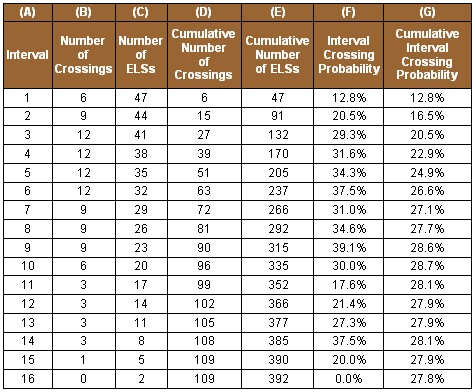The number of crossings shown in Column (B) is the same as what is displayed in the last column of Table 3A and Table 3B above. The total number of ELSs possible for each given interval (i) is given by Formula 1C.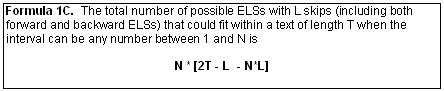For each interval, the cumulative number of crossings in Column (D) was calculated by summing the numbers in Column (B) for that interval and all smaller intervals. For example, the cumulative number of crossings for interval 3 is the sum of the number of crossings for intervals 3, 2 and 1, or 6 + 9 + 12 = 27. Similarly, for each interval, the cumulative number of ELSs in Column (E) was derived by summing the number of ELSs in Column (C) for that interval and all smaller intervals. So, for interval 3, the cumulative number of possible ELSs is 47 + 44 + 41 = 132. The interval crossing probabilities in Column (F) were determined by dividing the number of crossing ELSs from Column (B) by the total possible number of ELSs of that given interval within the entire text (Column (C)). As an example, consider all ELSs of interval 2. The above table tells us that there are 44 possible ELSs of interval 2 that can fit within the text of the first 50 letters of the Gettysburg Address. Of those 44, only nine, or 20.5%, cross the section of text covering spaces 30 through 32. Notice that the cumulative overlap probabilities start at fairly low percentages and increase up to around 28%. This is reflective of the fact that ELSs with greater intervals are more likely to cross any given section of text than are ELSs with very small intervals — such as 1, 2 or 3. We started this appendix with the objective of calculating the probability of a given ELS, which only occurs once, of crossing a given section of text. We proceeded by presenting and developing a specific example where we determined this probability. In the next appendix, we will generalize the content of this appendix in order to derive algebraic formulas for this probability.

 Enjoy finding your own Bible codes. Bible code search software is available in our online store. Subscribe Free! By signing up to be a member of The Isaac Newton Bible Research Society, you will have access to more than fifteen years of research by our team of Bible code researchers. Sign up to be a member today.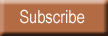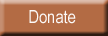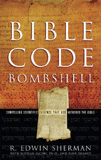Bombshell examines two massive, recently discovered clusters of codes in the Hebrew Old Testament. To read more about Bombshell, click here, or click below to order from Amazon today!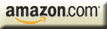| HOME | SUBSCRIBERS ONLY | OUR PURPOSE | FREE SAMPLES | CURRENT | | FAQs | ABOUT BIBLE CODES | SUBSCRIBE | CONTACT | Copyright © 2016 BibleCodeDigest.com